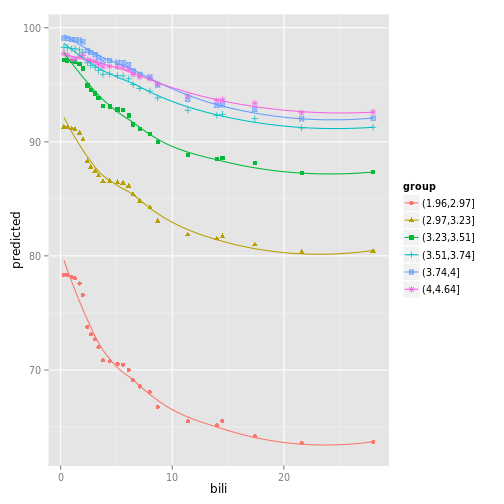# Data structures for stratified partial coplots

``````# Load the forest
data(rfsrc_pbc, package="ggRandomForests")

# Create the variable plot.
ggvar <- gg_variable(rfsrc_pbc, time = 1)

# Find intervals with similar number of observations.
copper_cts <-quantile_pts(ggvar\$copper, groups = 6, intervals = TRUE)

# Create the conditional groups and add to the gg_variable object
copper_grp <- cut(ggvar\$copper, breaks = copper_cts)

## Not run:
##D ## We would run this, but it's expensive
##D partial_coplot_pbc <- gg_partial_coplot(rfsrc_pbc, xvar = "bili",
##D                                          groups = copper_grp,
##D                                          surv_type = "surv",
##D                                          time = 1,
##D                                          show.plots = FALSE)
## End(Not run)
## so load the cached set
data(partial_coplot_pbc, package="ggRandomForests")

# Partial coplot
plot(partial_coplot_pbc, se = FALSE)``````
``````## Warning: The plyr::rename operation has created duplicates for the
## following name(s): (`se`)``````Updated